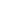Flat 50% off

Ends in

• JEE Main
•• Mathematics
• Back to Doubt Clearing

## Please give the solution for this questionIf an angle between the line, $\frac {x + 1}{2} = \frac {y - 2}{1} = \frac {z - 3}{-2}$ and the plane, $x-2 y-\mathrm{k} z=3$is $\cos ^{-1}\left(\frac{2 \sqrt{2}}{3}\right)$, then a value of $k$ is :(a) $\sqrt{\frac{5}{3}}$(b) $\sqrt{\frac{3}{5}}$(c) $-\frac{3}{5}$(d) $-\frac{5}{3}$

### Asked By K V HARSHA VARDHAN REDDY

Updated Thu, 23 May 2019 02:50 pm